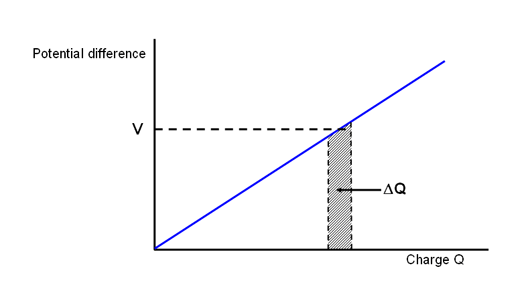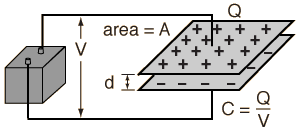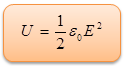Click to Chat

1800-1023-196

+91-120-4616500

CART 0

• 0

MY CART (5)

Use Coupon: CART20 and get 20% off on all online Study Material

ITEM
DETAILS
MRP
DISCOUNT
FINAL PRICE
Total Price: Rs.

There are no items in this cart.
Continue Shopping• Complete Physics Course - Class 11
• OFFERED PRICE: Rs. 2,968
• View Details

Energy Stored in a Capacitor

Table of Content

Formulae and results based on the energy stored in capacitor

Energy Density of a Capacitor

Energy stored in a group of Capacitors

Related ResourcesConsider a capacitor of capacity C. When a certain charge is given to it, it is raised through a certain potential. In order to add more charge to it, the new installment of charge has to be brought against the force of repulsion due to the charge already present upon it. Therefore, some work has to be done by the external agency.

The addition of new charge raises, further, the potential of the capacitor. Since, the additional installments of charge brought to the capacitor are against gradually increasing potential, so greater amount of work has to be done. The total work done to increase the charge on a capacitor from zero to a desired value gets stored in it and is known as the potential energy of the capacitor.A capacitor is basically a two terminal electrical component used for storage of energy electrostatically in an electric field. A large variety of capacitors are available but one thing that is common among all is that each capacitor contains at least two electrical conductors which are separated or partitioned by a dielectric insulator. One of the most commonly used capacitor consists of metal foils with a thin layer of insulating film in between.

The capacitance is at its peak when there is a thin separation between the big areas of conductor and it is due to this that the capacitor conductors are also called plates.

Formulae and Results based on the Energy Stored in Capacitor

We consider the case of charging a parallel plate capacitor which is originally uncharged. We shift a charge Q from one plate to another thus resulting in the former plate having a charge -Q and the later with charge +Q. once some electric charge has been transferred it leads to the development of some electric field which prohibits the transfer of any further charge. Hence, if we wish to charge the capacitor completely we need to work against this field and this work performed will constitute the energy stored in the capacitor. Let us see how this energy is calculated:Potential energy of a capacitor is defined as the amount of work done to charge the capacitor.

Let ‘q’ and ‘v’ be the instantaneous charge and the potential, respectively, of the capacitor during the process of charging.

Then, v = q/c

Now, if a charge ‘dq’ be brought from infinity and given to the capacitor and ‘dW’ is the work done in doing so, then

dW = v.dq     or dW = (q/c) .dq

Total work W required to charge the capacitor with a charge Q can be obtained by integrating the above equation between the limits 0 and Q.

So,

Or, W = 1/C[(Q2/2) – (0/2)]

W = ½ (Q2/C)               …... (1)

If V = final potential of the capacitor, then Q = CV

So, W = ½ [(CV)2/C]

Or, W = ½ CV2               …... (2)

But, C = Q/V

Substituting for ‘C” in equation (1), we get,

W = ½ [Q2/(Q/V)]…... (3)

Equation (1), (2) and (3) give the potential energy of the capacitor or the energy stored in the capacitor.

Therefore, the energy stored in the condenser resides in the electric field.

From the above observation we conclude that,

(1) The energy stored in a capacitor is proportional to the capacitance.

(2) The energy stored in a capacitor is proportional to the square of the voltage across the capacitor

(3) Since the square of the voltage appears in the energy formula, the energy stored is always positive.

(4) You can't have a negative amount of energy in the capacitor.

(5) That means you can put energy into the capacitor, and you can take it out, but you can't take out more than you put in.

Refer this simulation for Battery

Battery: A battery is a device with separated charges. When a wire connects the ends it channels the e-field between the ends. The e-field propels the charges along the wire. This animation is sparse. It accompanies a lecture. It starts with a charge and its e-field. Then it shows how the charge changes in proximity to the opposite charge. Next the charges are encased in a shell. This is the battery. Finally, a wire channels the e-field and propels the charge along the wire.

Energy Density of a CapacitorFor a parallel plate condenser,

C = ε0A/d

If ‘E’ is the strength of the field in between the two plates,

E = σ/ε0  or  σ =ε0E

Since Q = Aσ

So, Q = Aε0E

Substituting for Q and C in equation (1),

W = ½ [(Aε0E)2/(ε0A/d)]

= ½ A2ε02E2 (d/ε0A)

But, Ad = v (volume of space in between the two plates)

So, W = ½ ε0E2v           …... (4)

Energy stored in a capacitor per unit volume is called the energy density of the capacitor.

Thus, Energy density (U) = W/Volume = (½ ε0E2v) / v = ½ ε0E2

So, Energy Denssity,Thus, it is clear that the energy density of a capacitor is independent of the area of plates of distance between them so long the value of E does not change.

Equation (4) has been derived for a parallel plate condenser, but it is true for a condenser.

If Q = 0     or     V = 0

From equation (3), W = 0

Putting W = 0, in equation (4), E = 0

Thus, it is clear from equation (4), that values of E increases due to the increase in the energy stored in the condenser.

Therefore, the energy stored in the condenser resides in the electric field.

Energy Stored in a group of Capacitors

Case (i) Energy stored in a series combination of capacitors

Let  a charge ‘q’ be given to a group of capacitors of capacities C1, C2, and C3 respectively connected in series with each other. Since they are in series, each will get charged with the charge q. If ‘C’ is the net capacity of the combination,

1/C = 1/C1+ 1/C2+1/C3

Total energy W stored in the combination is

W = ½ (q2/C) = ½ q2[1/C1+ 1/C2+1/C3]

W = ½ (q2/C1) + ½ (q2/C2) + ½ (q2/C3)

Or, W = W1 +W2 +W3

Where W1, W2 and W3 are the energies stored in the three constituent capacitors of the combination.

Thus, net energy stored in the combination is equal to the sum of energies stored in the component capacitors.

Case (ii) Energy stored in a parallel combination of capacitors

When a number of capacitors having capacities C1,C2 and C3 are connected in parallel, they get charged to same potential V. If ‘C’ is the net capacity of the combination,

C = C1+C2+C3

Total energy W, stored in the combination is,

W = ½ CV2 or W = ½ (C1+C2+C3) V2

Or, W = ½ C1V2 + ½ C2V2 + ½ C3V2

Or, W = W1+W2+W3

Again we find that the net energy stored in the combination is equal to sum of energies stored in the component of capacitors.

View the following video for more on capacitorsThe energy stored in a capacitor is proportional to the capacitance

The energy stored is also proportional to the square of the voltage across the capacitor

From the last point it becomes obvious that the energy stored is always positive.

This may also be interpreted as it is possible to put in energy into the capacitor and it can also be taken out. But since the energy cannot be negative so the energy taken out cannot exceed the amount put in.

Power in to the capacitor can be negative.

Voltage can be positive while current is negative.

You could charge a capacitor by putting a battery across the capacitor. Then, if you placed a resistor across the capacitor, charge would leave the capacitor - current would flow out of the capacitor - and the energy in the capacitor would leave the capacitor only to become heat energy in the resistor.

When energy leaves the capacitor, power is negative.

When you use capacitors in a circuit and you analyze the circuit you need to be careful about sign conventions as defined in the illustration we used above - which is repeated here.

Problem:

A parallel plate capacitor consists of two metal plates, each of area A = 150 cm2, separated by a vacuum gap d = 0.60 cm thick. What is the capacitance of this device? What potential difference must be applied between the plates if the capacitor is to hold a charge of magnitude Q = 1.00 10-3 µC on each plate?

Solution:

Making use of formula C = ε0A/d, the capacitance C is given by

C = ε0A/d = (8.8510-12) (15010-4)/(0.610-2)

= 2.2110-11 = 22.1 pF

The voltage difference V between the plates and the magnitude of the charge Q  stored on each plate are related to C = Q/V, or V = Q/C. Hence, if Q = 1.0010-3µC, then,

V = (1.0010-9)/(2.2110-11) = 45.2 V

From the above observation we conclude that, the potential difference will be 45.2 V.

Problem (IIT 1983) :

Two identical parallel plate capacitors connected to a battery with the switch S closed. The switch is now opened and the free space between the plates of the capacitors is filled with a dielectric of dielectric constant (or relative permittivity) 3. Find the ratio of the total electrostatic energy stored in both capacitors before and after the introduction of dielectric.

Solution:

When the switch ‘S’ is closed both the capacitors A and B are at same potential V. Since they have same capacities also, so charge qA and qB on both of them is

qA = qB = CV

Total energy of the system E1

Or, E1 = ½ CV2 + ½ CV2 = CV2    …... (1)

When switch S is opened, both are still at same potential. As the dielectric of K = 3 is introduced in both of them, their capacities change from C to C' such that,

C' = KC = 3C

Now A is connected to source while B is not. Therefore, potential of A remains at V while that of B changes to V'.

V' = q/C' = q/3C = V/3

Total energy E2 of the system in second case is

E2  = ½ C'V2 + ½ C'V'2

= ½ (3C)V2 + ½ (3C) (V/3)2

= 3/2 (CV2) + (1/6) CV2

= CV2 (3/2 + 1/6)

= 5/3 CV2

Thus, E1/E2 = (CV2)/(5/3 CV2) = 3/5

Thus, the ratio of the total electrostatic energy stored in both capacitors before and after the introduction of dielectric would be 3/5.

Problem:

An air-filled parallel plate capacitor has a capacitance of 5.0 pF. A potential of 100V is applied across the plates, which are 1.0 cm apart, using a storage battery. What is the energy stored in the capacitor? Suppose that the battery is disconnected, and the plates are moved until they are 2.0 cm apart. What now is the energy stored in the capacitor? Suppose, instead, that the battery is left connected, and the plates are again moved until they are 2.0 cm apart. What is the energy stored in the capacitor in this case?

Solution:

The initial energy stored in the capacitor is

W = ½ CV2

= [(510-12) (100)2]/2

= 2.510-8 J

When the spacing between the plates is doubled, the capacitance of the capacitor is halved to 2.5 pF. If the battery is disconnected then this process takes place at constant charge Q . Thus, it follows from the formula

W = Q2/2C

that the energy stored in the capacitor doubles. So, the new energy is 5.010-8 J. Incidentally, the increased energy of the capacitor is accounted for by the work done in pulling the capacitor plates apart (since these plates are oppositely charged, they attract one another).

If the battery is left connected, then the capacitance is still halved, but now the process takes place at constant voltage V. It follows from the formula

W = CV2/2

that the energy stored in the capacitor is halved. So, the new energy is1.2510-8 J. Incidentally, the energy lost by the capacitor is given to the battery (in effect, it goes to re-charging the battery). Likewise, the work done in pulling the plates apart is also given to the battery.Question 1

The energy in a capacitor can be stored in the form of

(a) ½ CV2             (b) 2 CV2

(c) CV2                  (d) ½ CV

Question 2

One micro farad of capacitance is equal to the

(a) 10-2 F           (b) 10-3 F

(c) 10-4 F          (d) 10-6 F

Question 3

The ability of capacitor to store the charge is measured as its

(a) performance        (b) resistance

(c) capacitance        (d) durability

Question 4

Capacitor is used to store the electric

(a) charge          (b) energy

(c) voltage          (d) both a and bQ.1
Q.2
Q.3
Q.4

a

d

c

b

Related Resources

You might like to refer Capacitors.

For getting an idea of the type of questions asked, refer the  Previous Year Question Papers.

To read more, Buy study material of Electrostatics comprising study notes, revision notes, video lectures, previous year solved questions etc. Also browse for more study materials on Physics here.### Course Features

• 101 Video Lectures
• Revision Notes
• Previous Year Papers
• Mind Map
• Study Planner
• NCERT Solutions
• Discussion Forum
• Test paper with Video Solution